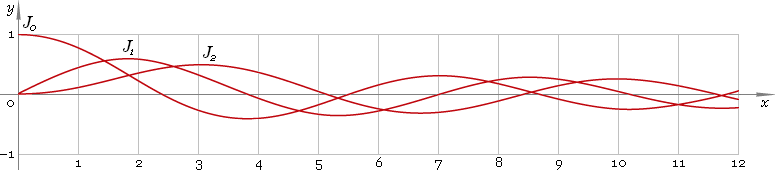The Art of Mathematics

# J — Bessel function of the first kind

## 1. Definition

By definition Bessel function is solution of the Bessel equation

z2 w′′ + z w′ + (z2 − ν2) w = 0

As second order equation it has two solutions, first of which has no singularity at 0 and is called Bessel function of the first kind — Jν. Parameter ν is called order of the function.

Second solution has singularity at 0 and is called Bessel function of the second kind — Yν.

## 2. Graph

Bessel functions of the first kind defined everywhere on the real axis, so their domain is (−∞, +∞). Graph of the first three representatives of the first kind Bessel function family depicted below — fig. 1.Fig. 1. Graphs of the Bessel functions of the first kind y = J0(x), y = J1(x) and y = J2(x).

## 3. Identities

Next order recurrence:

Jν+1(z) = 2ν /z Jν(z) − Jν−1(z)

Negative argument:

Jν(−z) = eiπν Jν(z) = [cos(πν) + i sin(πν)] Jν(z)

For the case of integer order ν=n the negative argument identity can be simplified down to:

Jn(−z) = (−1)n Jn(z)

and for the case of half-integer order ν=n+1/2 the identity can be simplified down to:

Jn+1/2(−z) = i (−1)n Jn+1/2(z)

Reflection — negative order:

J−ν(z) = cos(πν) Jν(z) − sin(πν) Yν(z)

For the case of integer order ν=n reflection identity can be simplified down to:

J−n(z) = (−1)n Jn(z)

and for case of half-integer order ν=n+1/2 the identity can be simplified down to:

J−n−1/2(z) = (−1)n+1 Yn+1/2(z)

## 4. Derivative

Bessel function of the first kind derivative:

J′ν(z) = Jν−1(z) − ν Jν(z) /z J′ν(z) = ν Jν(z) /z − Jν+1(z) J′ν(z) = [Jν−1(z) − Jν+1(z)] /2

In particular:

J′0(z) = −J1(z)

## 5. Interface

Bessel function call looks like

``J(ν, z);``

where ν is the function real order, and z — function complex argument.

## 6. How to use

To calculate Bessel function of the first kind of the 0 order of the number:

``J(0, −1.5+i);``

To get Bessel function of the first kind of the 1.2 order of the current result:

``J(1.2, rslt);``

To get Bessel function of the first kind of the 2.5 order of the number z in calculator memory:

``J(2.5, mem[z]);``

## 7. Support

Bessel function of the first kind of real order and complex argument is supported in professional version of the Librow calculator.